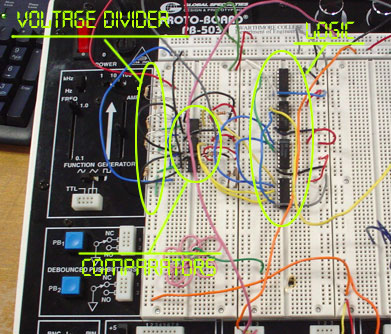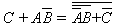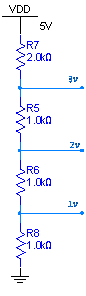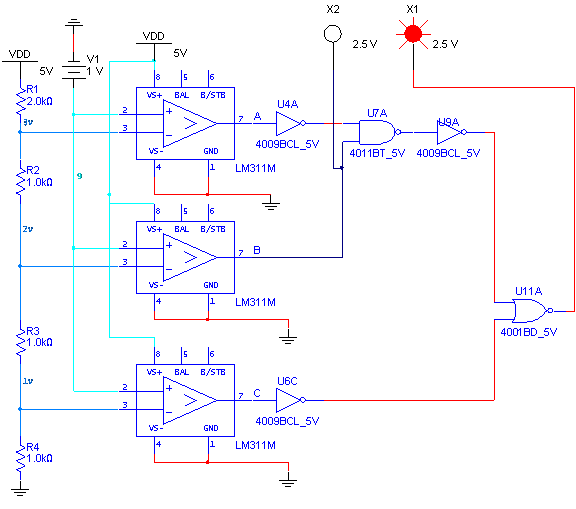E72 Electronic Circuit Applications - Lab 4
Digital Electronics
in partnership with mas chano
11.01.2005click on image for larger version of our circuit

 abstract.

In this lab we created a simple digital circuit using logic gates and comparators. Given a signal (Vsig) that varies between 0V and 5V, we designed the circuitry that outputs

00 if Vsig< 1V;

01 if 1 ≤ Vsig < 2V;

10 if 2 ≤ Vsig < 3V;

11 if 3 ≤ Vsig.

 procedure .

 digital circuit .

Let's represent the outputs of the comparators A, B and C. Please see figure 2 to see which letters correspond to which comparator.

Then the output for bit 1 (leftmost bit) is B. This is true because if the output is greater than 2V, the 1st bit is always on.

The output for bit 2 (rightmost bit) is. Namely, the 2nd bit is on if the test voltage is between 1 and 2V, or if the test voltage is higher than 3V.

For our comparators we used the voltage divider seen in figure 1.Figure 1 . The voltage divider that provides the reference voltages to the comparators.

We implemented our circuit as seen in figure 2.Figure 2 . Logic Circuit for this lab. The lights indicate on/off. (in this specific figure the input signal is 1V and the output is, accordingly, 01)

For our circuit, we used three LM311 comparators, one DM7404N hex-inverter, one DM7402 quad 2-input NOR and a DM7400 quad 2-input NAND.
We confirmed our cicruit with a real implementation.

You can see the videos of the working circuit at http://engin/~mchano1/temporary/72/72labfiles.zip  [zip file ~40mb]

 what we learned.

This lab was very useful in terms of integrating analog and digital components together. It also helped us with testing and understanding where the roots of our problems can be.

 problems encountered.

Although our design was solid, we had a lot of problems with the individual components. We usually assumed the components worked well and assumed there was a mistake with our design, and made some changes. However, eventually just replacing faulty components solved our problems. While working on this lab, one should make sure each component works fine before integrating it in the whole implementation.